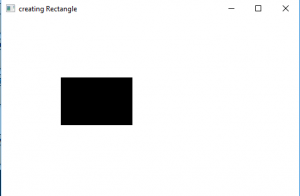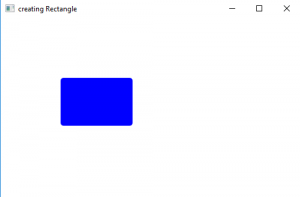# JavaFX | Rectangle and Rounded Rectangle with examples

Rectangle class is a part of JavaFX. Rectangle class creates a rectangle with specified width and height and position.
By default Rectangle has sharp corners but the edges can be rounded by applying a arc height and width.

Constructor :

1. Rectangle() : creates a empty instance of rectangle
2. Rectangle(double w, double h) : creates a rectangle with a specified width and height
3. Rectangle(double x, double y, double w, double h) : creates a rectangle with a specified width and height and position
4. Rectangle(double w, double h, Paint f) : creates a rectangle with a specified width and height and fill

Commonly used methods

method explanation
getArcHeight() returns the arc height of the rectangle
getArcWidth() returns the arc width of the rectangle
getHeight() returns the height of the rectangle
getWidth() retuns the width of the rectangle
getX() Gets the value of the property x.
getY() Gets the value of the property y.
setArcHeight(double v) sets the arc height of the rectangle
setArcWidth(double v) sets the arc width of the rectangle
setHeight(double value) sets the height of the rectangle
setWidth(double value) sets the width of rectangle
setX(double value) set the x coordinate of position of reactangle
setY(double value) set the y coordinate of position of reactangle

Sample program to illustrate the use of Rectangle class

This program creates a Rectangle indicated by the name rectangle( the coordinates of the position and the height and width is passed as arguments). The Rectangle will be created inside a scene, which in turn will be hosted inside a stage. The function setTitle() is used to provide title to the stage. Then a Group is created, and the circle is attached.The group is attached to the scene. Finally, the show() method is called to display the final results.
Program to create a rectangle and add it to the scene

 `// Java Program to create a rectangle and add it to the scene ` `import` `javafx.application.Application; ` `import` `javafx.scene.Scene; ` `import` `javafx.scene.control.Button; ` `import` `javafx.scene.layout.*; ` `import` `javafx.scene.paint.Color; ` `import` `javafx.scene.shape.Rectangle; ` `import` `javafx.scene.control.*; ` `import` `javafx.stage.Stage; ` ` `  `import` `javafx.scene.Group; ` `public` `class` `Rectangle_0 ``extends` `Application { ` ` `  `    ``// launch the application ` `    ``public` `void` `start(Stage stage) ` `    ``{ ` `        ``// set title for the stage ` `        ``stage.setTitle(``"creating Rectangle"``); ` ` `  `        ``// create a rectangle ` `        ``Rectangle rectangle = ``new` `Rectangle(``100``.0d, ``100``.0d, ``120``.0d, ``80``.0d); ` ` `  `        ``// create a Group ` `        ``Group group = ``new` `Group(rectangle); ` ` `  `        ``// create a scene ` `        ``Scene scene = ``new` `Scene(group, ``500``, ``300``); ` ` `  `        ``// set the scene ` `        ``stage.setScene(scene); ` ` `  `        ``stage.show(); ` `    ``} ` ` `  `    ``public` `static` `void` `main(String args[]) ` `    ``{ ` `        ``// launch the application ` `        ``launch(args); ` `    ``} ` `} `

OutputProgram to create a rounded rectangle and set a fill and add it to the scene

This program creates a Rectangle indicated by the name rectangle( the coordinates of the position and the height and width is passed as arguments). The rounded corners will be set using setArcHeight() and setArcWidth() function .The fill for the rectangle will be set using setFill() function. The Rectangle will be created inside a scene, which in turn will be hosted inside a stage. The function setTitle() is used to provide title to the stage. Then a Group is created, and the circle is attached.The group is attached to the scene. Finally, the show() method is called to display the final results.

 `// Java Program to create a rounded rectangle ` `// and set a fill and add it to the scene ` `import` `javafx.application.Application; ` `import` `javafx.scene.Scene; ` `import` `javafx.scene.control.Button; ` `import` `javafx.scene.layout.*; ` `import` `javafx.scene.paint.Color; ` `import` `javafx.scene.shape.Rectangle; ` `import` `javafx.scene.control.*; ` `import` `javafx.stage.Stage; ` ` `  `import` `javafx.scene.Group; ` `public` `class` `Rectangle_1 ``extends` `Application { ` ` `  `    ``// launch the application ` `    ``public` `void` `start(Stage stage) ` `    ``{ ` `        ``// set title for the stage ` `        ``stage.setTitle(``"creating Rectangle"``); ` ` `  `        ``// create a rectangle ` `        ``Rectangle rectangle = ``new` `Rectangle(``100``.0d, ``100``.0d, ``120``.0d, ``80``.0d); ` ` `  `        ``// set fill for rectangle ` `        ``rectangle.setFill(Color.BLUE); ` ` `  `        ``// set rounded corners ` `        ``rectangle.setArcHeight(``10``.0d); ` `        ``rectangle.setArcWidth(``10``.0d); ` ` `  `        ``// create a Group ` `        ``Group group = ``new` `Group(rectangle); ` ` `  `        ``// create a scene ` `        ``Scene scene = ``new` `Scene(group, ``500``, ``300``); ` ` `  `        ``// set the scene ` `        ``stage.setScene(scene); ` ` `  `        ``stage.show(); ` `    ``} ` ` `  `    ``public` `static` `void` `main(String args[]) ` `    ``{ ` `        ``// launch the application ` `        ``launch(args); ` `    ``} ` `} `

Output:Note: The following programs might not run in an online IDE please use an offline compiler
Reference:
https://docs.oracle.com/javase/8/javafx/api/javafx/scene/shape/Rectangle.html

Attention reader! Don’t stop learning now. Get hold of all the important DSA concepts with the DSA Self Paced Course at a student-friendly price and become industry ready.

My Personal Notes arrow_drop_upCheck out this Author's contributed articles.

If you like GeeksforGeeks and would like to contribute, you can also write an article using contribute.geeksforgeeks.org or mail your article to contribute@geeksforgeeks.org. See your article appearing on the GeeksforGeeks main page and help other Geeks.

Please Improve this article if you find anything incorrect by clicking on the "Improve Article" button below.

Improved By : ManasChhabra2

Article Tags :
Practice Tags :

Be the First to upvote.

Please write to us at contribute@geeksforgeeks.org to report any issue with the above content.